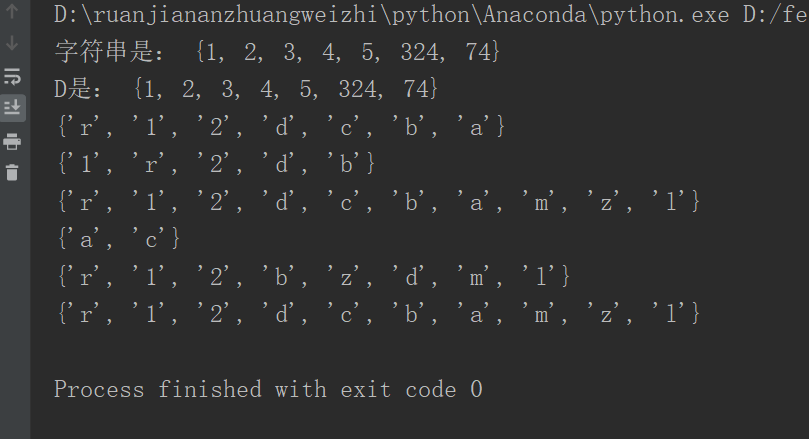出租广告位,需要合作请联系站长

+关注

2021-02(23)

2021-03(43)

python集合的操作

if __name__=="__main__":
b=set("alacazam")
list1=[1,3,4,324,5,74,2]
list2=[1,3,4,324,5,74,2,235,5643,26,85,32]
B=set()

A=set()
A.update(list1)
B.update(list2)
print("字符串是：",A)
D=A&B
print("D是：",D)
print(a)
c=a-b #集合a包含而集合b没有的元素
d=a|b#集合a和集合b的并集
e=a&b #集合a和集合b的交集
g=a.union(b)
f=a^b #不同时包含在集合a和集合b的元素
print(c)
print(d)
print(e)
print(f)
print(g)25 0

pdf(new) 更多>